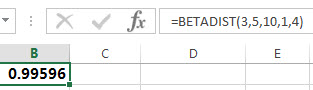This post will guide you how to use the BETADIST function with syntax and examples in Microsoft excel.

### Description

The Excel BETADIST function returns the cumulative beta probability density function. So you can use the BETADIST function to calculate the cumulative beta distribution for a given set of parameters in Excel. And the beta distribution is used to study variation in the percentage of something across samples. And this function has been replaced by BETA.DIST function.

The BETADIST function is a build-in function in Microsoft Excel and it is categorized as a Statistical Function.

The BETADIST function is available in Excel 2016, Excel 2013, Excel 2010, Excel 2007, Excel 2003, Excel XP, Excel 2000, Excel 2011 for Mac.

### Syntax

The syntax of the BETADIST function is as below:

`= BETADIST(x, alpha, beta, [A], [B])`

Where the BETA.DIST function arguments are:

• X -This is a required argument.  The value that is between A and B at which the function is to be calculated.
• Alpha – This is a required argument.  It is a parameter of the distribution.
• Beta – This is a required argument.  It is a parameter of the distribution.
• A – This is an optional argument. A lower bound to the interval of x. If it is omitted, it will be set the default value 0.
• B – This is an optional argument. A upper bound to the interval of x. If it is omitted, it will be set the default value 1.

Note:

• If any argument is non-numeric, the BETADIST function will return the #VALUE! Error.
• If the Alpha or Beta argument is smaller than or equal to 0, then the BETADIST function will return #NUM! Error.
• If the argument X is small than A and greater than B, the BETADIST function will Return #NUM! Error.

The below examples will show you how to use Excel BETADIST Function to calculate the cumulative beta distribution.

1# to calculate the cumulative beta probability density function for a supplied set of parameters, using the following formula:

`=BETADIST(3,5,10,1,4)`### Related Functions

• Excel BETA.DIST function
The Excel BETA.DIST function can be used to calculate the cumulative beta distribution or beta probability density function.The syntax of the BETA.DIST function is as below:= BETA.DIST(x, alpha, beta, cumulative, [A], [B]).…
• Excel BETA.INV function
The Excel BETA.INV function returns the inverse of the beta cumulative probability density function. So if the probability is equal to BETA.DIST(x,…TRUE), then the BETA.INV(probability,…) will return x.The syntax of the BETA.INV function is as below:= BETA.INV(probability, alpha, beta, [A], [B]).…
• Excel BETAINV function
The Excel BETAINV function returns the inverse of the beta cumulative probability density function. The syntax of the BETAINV function is as below:= BETAINV(probability, alpha, beta, [A], [B]).…
• Excel BINOM.DIST function
The Excel BINOM.DIST function returns the individual term binomial distribution probability. The syntax of the BINOM.DIST function is as below:= BINOM.DIST(number_s, trials, probability_s, cumulative).…
Related Posts

Excel DVARP Function

This post will guide you how to use Excel DVARP function with syntax and examples in Microsoft excel. Description The Excel DVARP Function will get the variance of a population based on the entire population of numbers in a column ...

Excel DVAR Function

This post will guide you how to use Excel DVAR function with syntax and examples in Microsoft excel. Description The Excel DVAR Function will get the variance of a population based on a sample of numbers in a column in ...

Excel DSUM Function

This post will guide you how to use Excel DSUM function with syntax and examples in Microsoft excel. Description The Excel DSUM Function will add the numbers in a column or database that meets a given criteria. And so you ...

Excel DSTDEVP Function

This post will guide you how to use Excel DSTDEVP function with syntax and examples in Microsoft excel. Description The Excel DSTDEVP returns the standard deviation of a population based on the entire population of numbers in a column or ...

Excel DSTDEV Function

This post will guide you how to use Excel DSTDEV function with syntax and examples in Microsoft excel. Description The Excel DSTDEV returns the standard deviation of a population based on a sample of numbers in a column or database ...

Excel DMIN Function

This post will guide you how to use Excel DMIN function with syntax and examples in Microsoft excel. Description The Excel DMIN returns the minimum value from a database or a column of a list that matches the specified conditions. ...

Excel DPRODUCT Function

This post will guide you how to use Excel DPRODUCT function with syntax and examples in Microsoft excel. Description The Excel DPRODUCT returns the product of values from a set of records that match criteria that you specify. The DPRODUCT ...

Excel XIRR Function

This post will guide you how to use Excel XIRR function with syntax and examples in Microsoft excel. Description The Excel XIRR function returns the internal rate of return for a series of cash flows that is not necessarily periodic. ...

Excel VDB Function

This post will guide you how to use Excel VDB function with syntax and examples in Microsoft excel. Description The Excel VDB function calculates the depreciation of an asset for a specified period based on the double declining balance method. ...

Excel SYD Function

This post will guide you how to use Excel SYD function with syntax and examples in Microsoft excel. Description The Excel SYD function calculates the sum-of-years’digits depreciation of an asset for a specified period. And it will return a numeric ...

Sidebar